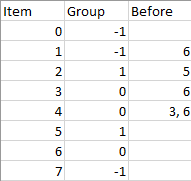# 1203. Sort Items by Groups Respecting Dependencies

There are `n` items each belonging to zero or one of `m` groups where `group[i]` is the group that the `i`-th item belongs to and it’s equal to `-1` if the `i`-th item belongs to no group. The items and the groups are zero indexed. A group can have no item belonging to it.

Return a sorted list of the items such that:

• The items that belong to the same group are next to each other in the sorted list.
• There are some relations between these items where `beforeItems[i]` is a list containing all the items that should come before the `i`-th item in the sorted array (to the left of the `i`-th item).

Return any solution if there is more than one solution and return an empty list if there is no solution.

Example 1:``````Input: n = 8, m = 2, group = [-1,-1,1,0,0,1,0,-1], beforeItems = [[],,,,[3,6],[],[],[]]
Output: [6,3,4,1,5,2,0,7]
``````

Example 2:

``````Input: n = 8, m = 2, group = [-1,-1,1,0,0,1,0,-1], beforeItems = [[],,,,,[],,[]]
Output: []
Explanation: This is the same as example 1 except that 4 needs to be before 6 in the sorted list.
``````

Constraints:

• `1 <= m <= n <= 3 * 104`
• `group.length == beforeItems.length == n`
• `-1 <= group[i] <= m - 1`
• `0 <= beforeItems[i].length <= n - 1`
• `0 <= beforeItems[i][j] <= n - 1`
• `i != beforeItems[i][j]`
• `beforeItems[i] `does not contain duplicates elements.

``````class Solution {
public:
vector<int> sortItems(int n, int m, vector<int>& group, vector<vector<int>>& beforeItems) {
for (int i = 0; i < n; ++i) {
if (group[i] == -1) group[i] = m++;
}
vector<unordered_set<int>> graphGroup(m), graphItem(n);
vector<int> inGroup(m), inItem(n), res;
for (int i = 0; i < n; ++i) {
int to_group = group[i];
for (int j : beforeItems[i]) {
int from_group = group[j];
if (from_group != to_group && !graphGroup[from_group].count(to_group)) {
graphGroup[from_group].insert(to_group);
++inGroup[to_group];
}
if (!graphItem[j].count(i)) {
graphItem[j].insert(i);
++inItem[i];
}
}
}
vector<int> group_sorted = helper(graphGroup, inGroup), item_sorted = helper(graphItem, inItem);
if (group_sorted.empty() || item_sorted.empty()) return {};
vector<vector<int>> group2item(m);
for (int item : item_sorted) {
group2item[group[item]].push_back(item);
}
for (int group_id : group_sorted) {
for (int item : group2item[group_id]) {
res.push_back(item);
}
}
return res;
}
vector<int> helper(vector<unordered_set<int>>& g, vector<int>& inDegree) {
vector<int> res;
queue<int> q;
for (int i = 0; i < inDegree.size(); ++i) {
if (inDegree[i] == 0) q.push(i);
}
while (!q.empty()) {
int t = q.front(); q.pop();
res.push_back(t);
for (int next : g[t]) {
if (--inDegree[next] == 0) {
q.push(next);
}
}
}
for (int i = 0; i < inDegree.size(); ++i) {
if (inDegree[i] > 0) return {};
}
return res;
}
};
``````

``````class Solution {
public:
vector<int> sortItems(int n, int m, vector<int>& group, vector<vector<int>>& beforeItems) {
vector<int> t, res(n), state(n + 2 * m);
vector<vector<int>> g(n + 2 * m);
for (int i = 0; i < n; ++i) {
if (group[i] != -1) {
g[n + group[i]].push_back(i);
g[i].push_back(n + m + group[i]);
}
for (int j : beforeItems[i]) {
if (group[i] != -1 && group[i] == group[j]) {
g[j].push_back(i);
} else {
int p = group[i] == -1 ? i : n + group[i];
int q = group[j] == -1 ? j : n + m + group[j];
g[q].push_back(p);
}
}
}
for (int i = (int)g.size() - 1; i >= 0; --i) {
if (!helper(g, i, state, t)) return {};
}
reverse(t.begin(), t.end());
copy_if(t.begin(), t.end(), res.begin(), [&](int i) {return i < n;});
return res;
}
bool helper(vector<vector<int>>& g, int i, vector<int>& state, vector<int>& res) {
if (state[i] != 0) return state[i] == 2;
state[i] = 1;
for (int next : g[i]) {
if (!helper(g, next, state, res)) return false;
}
state[i] = 2;
res.push_back(i);
return true;
}
};
``````

Github 同步地址:

https://github.com/grandyang/leetcode/issues/1203

https://leetcode.com/problems/sort-items-by-groups-respecting-dependencies/

https://leetcode.com/problems/sort-items-by-groups-respecting-dependencies/discuss/402945/C%2B%2B-with-picture-generic-topological-sort

https://leetcode.com/problems/sort-items-by-groups-respecting-dependencies/discuss/1106701/C%2B%2B-Two-level-topological-sort.-Peel-off-the-tricky-parts-then-do-normal-TopoSort.

LeetCode All in One 题目讲解汇总(持续更新中…)

 微信打赏Venmo 打赏×

Help us with donation